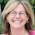## Monday, October 5, 2009

### Rounding Functions

Round and Round she goes................
I didn't realize how many different rounding functions there are. And, I assuming neither did you.
=Round, =Roundup() and =Rounddown() all round a number to a specified number of decimal places.
=Round(12,3456,1) returns a value of 12.3 since 1 decimal place was specified.
=Round(12.3456,2) return a value of 12.35 since 2 decimal places were specified.

RoundUp always rounds away from the Zero and RoundDown always rounds toward the Zero.
=Roundup(12.3456,1) returns a value of 12.4
=Rounddown(12.3456,1) returns a value of 12.3

Rounding to a Multiple
MRound(), Ceiling() and Floor() round numbers to a specified multiple.
These 3 functions can be used for rounding price points or time.

=MROUND(\$12.94,5) will round it to the nearest 5 increment and display as \$12.95
=MRound(\$12.94,.025) will round to the nearest .25 increment and display as \$13.00

Ceiling() and Floor() are traditionally used with dollar values. They will not round if the number is already a multiple of the number. For example, =Ceiling(3.4,1) will still display as 3.4 since 3.4 is already a multiple of 1.

1.2.